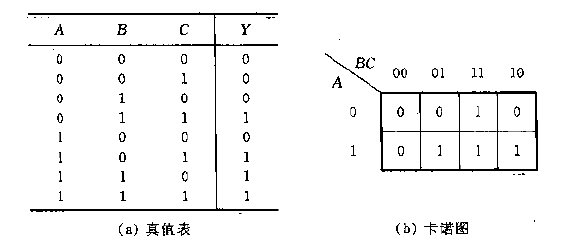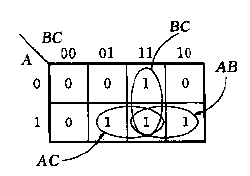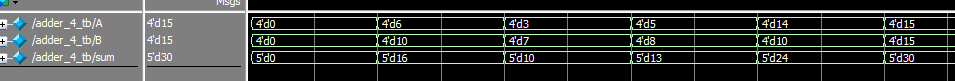# Verilog 学习笔记（6）——Verilog 语言基础（4）Contents

## 组合逻辑

### 卡诺图$$Y=\overline{A}BC+A\overline{B}C+AB\overline{C}+ABC$$

$$Y=AC+BC+AB$$

• 卡诺圈必须包含了所有最小项（即图中所有1）
• 卡诺圈只能是 1*1,1*2,2*2,2*4,4*4 （一般卡诺图最大画到4*4,因为再大的卡诺图无法保证全部相邻）
• 卡诺图最左和最右是相邻的，最上和最下是相邻的

### 组合逻辑电路分析

1. 将电路模块化
2. 写出各级输出与输入信号的逻辑关系
3. 化简逻辑函数
4. 用连续赋值语句搭建电路模块

### 组合电路设计

#### e.g.1

Ring or vibrate

module top_module (
input ring,
input vibrate_mode,
output ringer,       // Make sound
output motor         // Vibrate
);


always@(*)
if(vibrate_mode&ring)
begin
motor=1;
ringer=0;
end
else if(ring)
begin
motor=0;
ringer=1;
end
else
begin
motor=0;
ringer=0;
end


module top_module (
input ring,
input vibrate_mode,
output ringer,       // Make sound
output motor         // Vibrate
);

assign ringer=ring&(!vibrate_mode);
assign motor=ring&vibrate_mode;

endmodule


#### e.g.2

module adder_1(
input a,
input b,
input cin,
output sum,
output cout
);

assign {cout,sum} = a+b+cin;

endmodule


$$C_i=A_iB_i+A_iC_{i-1}+B_iC_{i-1}=A_iB_i+(A_i+B_i)C_{i-1}$$

$$C_0=P_0+Q_0C_{-1}\\ C_1=P_1+Q_1C_{0}=P_1+Q_1(P_0+Q_0C_{-1})=P_1+P_0Q_1+Q_0Q_1C_{-1}\\ C_2=P_2+P_1Q_2+P_0Q_1Q_2+Q_0Q_1Q_2C_{-1}\\ C_3=P_3+P_2Q_3+P_1Q_2Q_3+P_0Q_1Q_2Q_3+Q_0Q_1Q_2Q_3C_{-1}$$

module adder_1(
input a,
input b,
input cin,
output P,
output Q,
output sum
);

assign sum=a^b^cin;
assign P=a&b;
assign Q=a|b;

endmodule


module cpg(
input [3:0] P,
input [3:0] Q,
input cin,
output [3:0] cout
);

assign cout=P | Q&cin;
assign cout=P | Q&P | Q&Q&cin;
assign cout=P | Q&P | Q&Q&P | Q&Q&Q&cin;
assign cout=P | Q&P | Q&Q&P | Q&Q&Q&P | Q&Q&Q&Q&cin;

endmodule


module adder_4(
input [3:0] a,
input [3:0] b,
input cin,
output [4:0] sum
);

wire [3:0] P,Q;
wire [2:0] cout;

.a(a),
.b(b),
.cin(cin),
.P(P),
.Q(Q),
.sum(sum)
);

.a(a),
.b(b),
.cin(cout),
.P(P),
.Q(Q),
.sum(sum)
);

.a(a),
.b(b),
.cin(cout),
.P(P),
.Q(Q),
.sum(sum)
);

.a(a),
.b(b),
.cin(cout),
.P(P),
.Q(Q),
.sum(sum)
);

cpg cpg1(
.P(P),
.Q(Q),
.cin(cin),
.cout({sum,cout[3:0]})
);

endmodule


timescale 1ns/1ns

reg  [3:0]  A;
reg  [3:0]  B;
wire [4:0]  sum;

initial begin
A = 0;
B = 0;
#10;
A = 4'b0110;
B = 4'b1010;
#10;
A = 4'd3;
B = 4'd7;
#10;
A = 5;
B = 8;
#10;
A = 4'he;
B = 4'ha;
#10;
A = 4'hf;
B = 4'hf;
end

//initial begin
//    #100 \$finish;
//end

.a(A),
.b(B),
.cin(1'b0),
.sum(sum)
);

endmodule1.DylanOuO说道：